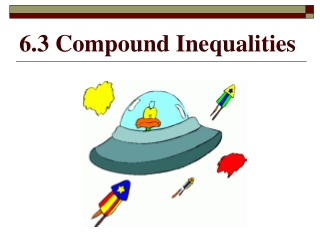# 6.3 Compound Inequalities - PowerPoint PPT PresentationDownload Presentation6.3 Compound Inequalities

6.3 Compound Inequalities
Download Presentation## 6.3 Compound Inequalities

- - - - - - - - - - - - - - - - - - - - - - - - - - - E N D - - - - - - - - - - - - - - - - - - - - - - - - - - -
##### Presentation Transcript

1. 6.3 Compound Inequalities

2. Learning Goal for Focus 2(HS.A-CED.A.1, 2 & 3, HS.A-REI.A.1, HS.A-REI.B.3): The student will create equations from multiple representations and solve linear equations and inequalities in one variable explaining the logic in each step.

3. Learn to Read… a< x <b • Read:x is between a and b a<x< b • Read:x is between a & b inclusive

4. Graph: 1< x < 5 Read as: ? x is between 1 and 5 0 1 2 3 4 5 6 7

5. Write the inequality x greater than 2 or x less than -1 x < -1 x > 2 Graph: -2 -1 0 1 2 3 *Remember: (closed circle) • when the number is included!

6. Solve Compound Inequalities and Graph: Solve like an equation, but now you have three parts! -9 < 6x + 3 ≤ 39 Subtract 3 from all three parts. -3 -3 -3 -12 < 6x≤ 36 Divide each part by 6. 6 6 6 -2 < x≤ 6 GRAPH: -3 -2 -1 0 1 2 3 4 5 6 7

7. Write the inequality, solve and then graph: Eight times a number x plus 4 is between -4 and 20. -4< 8x + 4 < 20 -4 -4-4 -8 < 8x < 16 -1 < x < 2 -2 -1 0 1 2 3

8. Use inequalities to solve the following problem. What are the restriction on the value of x in the triangle? Remember that two side of a triangle must add up to be greater than the third side. You must set up three inequalities! x 4 x + 4 > 6 x > 2 x + 6 > 4 x > -2 *length cannot be negative* 6 + 4 > x 10 > x 6 2 < x < 10

9. Practice: Graph 1. x> 4 and x < -2 2. -2 < x< 3 -3 -2 -1 0 1 2 3 4 5 -2 -1 0 1 2 3 Solve 2 + x> 3 3 < 2 - x < 5 x≥ 3 -3 < x < -1 5. Write an inequality for the following graph 2 3 4 5 6 7 8 9 10 4≤ x ≤ 8